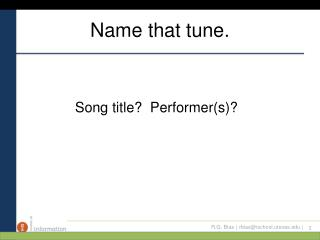DownloadDownload PresentationName that tune.

# Name that tune.

Télécharger la présentation## Name that tune.

- - - - - - - - - - - - - - - - - - - - - - - - - - - E N D - - - - - - - - - - - - - - - - - - - - - - - - - - -
##### Presentation Transcript

1. Name that tune.  Song title?  Performer(s)?

2. Scientific Method (continued) “Finding New Information” 4/11/2011

3. Objectives • I want you to understand z scores. “Standard scores.” • (Note – not standard deviation!) • Pencils down. I don’t want you to take notes – just get the concept. • Randolph – remember to take roll.

4. Which when?

5. So far . . . • . . . we’ve talked of summarizing ONE distribution of scores. • By ordering the scores. • By organizing them in graphs/tables/charts. • By calculating a measure of central tendency and a measure of dispersion. • What happens when we want to compare TWO distributions of scores?

6. “Now, why would I want to do that”? • Is your child taller or heavier? • Is this month’s SAT test any easier or harder than last month’s? • Is my 91 in my History class better than my 95 in my Math class? • Is the new library lay-out better than the old one? • Can more employees sign up, more quickly, for benefits with our new intranet site than with our old one? • Did my class perform better on the TAKS test than they did on the TAAS test?

7. Well? • COULD it be the case that your 91 in your History class is better than your 95 in your Math class? • How?

8. What if . . . • The mean in History was 50, and the mean in Digital Libraries Math was 99? • (What, besides the fact that everyone else is trying to drop the History class!) • So:

9. The Point • As I said last week, you need to know BOTH a measure of central tendency AND a measure of spread to understand a distribution. • BUT STILL, this can be convoluted . . . • “Well, daughter, how are you doing in school this semester”?

10. “Well, Mom . . . • “. . . I had a 91 on my History test but the mean was 50 and the standard deviation was 12. But I got a 95 on Math test, whereas the mean on that test was 99 with a standard deviation of 1.” • Of course, your mom’s reaction will be, “Just call home more often, dear.”

11. Wouldn’t it be nice . . . • . . . if there could be one score we could use for BOTH classes, for BOTH the TAKS test and the TAAS test, for BOTH your child’s height and weight? • There is – and it’s called the “standard score,” or “z score.”

12. Standard Score • z = (X - µ)/σ • “Hunh”? • Each score can be expressed as the number of standard deviations it is from the mean of its own distribution. • “Hunh”? • (X - µ) – This is how far the score is from the mean. (Note: Could be negative! No squaring, this time.) • Then divide by the SD to figure out how many SDs you are from the mean.

13. Z scores (cont’d.) • z = (X - µ)/σ • Notice, if your score (X) equals the mean, then z is, what? • If your score equals the mean PLUS one standard deviation, then z is, what? • If your score equals the mean MINUS one standard deviation, then z is, what?

14. An example

15. Let’s calculate σ – Test 1 – Mean = 61

16. Let’s calculate σ – Test 2= Mean = 83

17. So . . . z = (X - µ)/σ • Kris had a 76 on both tests. • Test 1 - µ = 61, σ = 9 • So her z score was (76-61)/9 or 15/9 or 1.67. So we say that Kris’s score was 1.67 standard deviations above the mean. Her z score was 1.67. • Test 2 - µ = 83, σ = 5.4 • So her z score was (76-83)/5.4 or -7/5.4 or –1.3. So we say that Kris’s score was 1.3 standard deviations BELOW the mean. Her z score was -1.3. • Given what I said earlier about two-thirds of the scores being within one standard deviation of the mean . . . . • Wouldn’t it be nice if we knew exactly how many . . . ?

18. z = (X - µ)/σ • If I tell you that the average IQ score is 100, and that the SD of IQ scores is 16, and that Bob’s IQ score is 2 SD above the mean, what’s Bob’s IQ? • If I tell you that your 75 was 1.5 standard deviations below the mean of a test that had a mean score of 90, what was the SD of that test?

19. z scores – table values • z = (X - µ)/σ • It is often the case that we want to know “What percentage of the scores are above (or below) a certain other score”? • Asked another way, “What is the area under the curve, beyond a certain point”? • THIS is why we calculate a z score, and the way we do it is with the z table.

20. Z distribution

21. Study Guide . . . • Later tonight, online.